# Manifold learning on handwritten digits: Locally Linear Embedding, Isomap…¶

We illustrate various embedding techniques on the digits dataset.

# Authors: Fabian Pedregosa <fabian.pedregosa@inria.fr>
#          Olivier Grisel <olivier.grisel@ensta.org>
#          Mathieu Blondel <mathieu@mblondel.org>
#          Gael Varoquaux
#          Guillaume Lemaitre <g.lemaitre58@gmail.com>
# License: BSD 3 clause (C) INRIA 2011


We will load the digits dataset and only use six first of the ten available classes.

from sklearn.datasets import load_digits

X, y = digits.data, digits.target
n_samples, n_features = X.shape
n_neighbors = 30


We can plot the first hundred digits from this data set.

import matplotlib.pyplot as plt

fig, axs = plt.subplots(nrows=10, ncols=10, figsize=(6, 6))
for idx, ax in enumerate(axs.ravel()):
ax.imshow(X[idx].reshape((8, 8)), cmap=plt.cm.binary)
ax.axis("off")
_ = fig.suptitle("A selection from the 64-dimensional digits dataset", fontsize=16)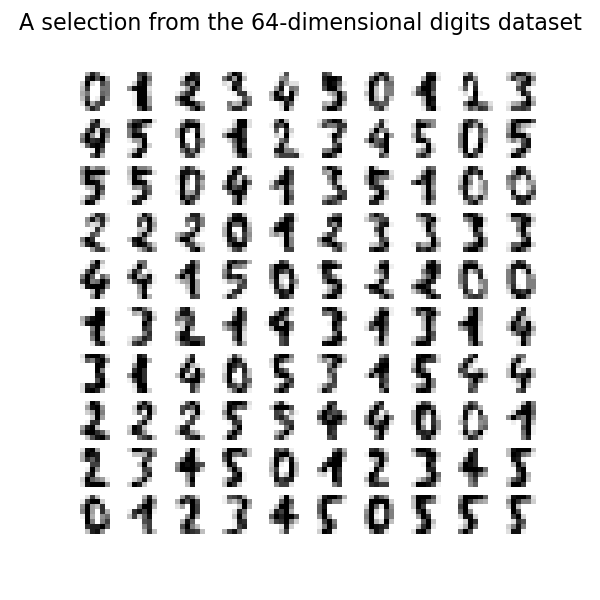## Helper function to plot embedding¶

Below, we will use different techniques to embed the digits dataset. We will plot the projection of the original data onto each embedding. It will allow us to check whether or digits are grouped together in the embedding space, or scattered across it.

import numpy as np
from matplotlib import offsetbox

from sklearn.preprocessing import MinMaxScaler

def plot_embedding(X, title):
_, ax = plt.subplots()
X = MinMaxScaler().fit_transform(X)

for digit in digits.target_names:
ax.scatter(
*X[y == digit].T,
marker=f"${digit}$",
s=60,
color=plt.cm.Dark2(digit),
alpha=0.425,
zorder=2,
)
shown_images = np.array([[1.0, 1.0]])  # just something big
for i in range(X.shape):
# plot every digit on the embedding
# show an annotation box for a group of digits
dist = np.sum((X[i] - shown_images) ** 2, 1)
if np.min(dist) < 4e-3:
# don't show points that are too close
continue
shown_images = np.concatenate([shown_images, [X[i]]], axis=0)
imagebox = offsetbox.AnnotationBbox(
offsetbox.OffsetImage(digits.images[i], cmap=plt.cm.gray_r), X[i]
)
imagebox.set(zorder=1)

ax.set_title(title)
ax.axis("off")


## Embedding techniques comparison¶

Below, we compare different techniques. However, there are a couple of things to note:

• the RandomTreesEmbedding is not technically a manifold embedding method, as it learn a high-dimensional representation on which we apply a dimensionality reduction method. However, it is often useful to cast a dataset into a representation in which the classes are linearly-separable.

• the LinearDiscriminantAnalysis and the NeighborhoodComponentsAnalysis, are supervised dimensionality reduction method, i.e. they make use of the provided labels, contrary to other methods.

• the TSNE is initialized with the embedding that is generated by PCA in this example. It ensures global stability of the embedding, i.e., the embedding does not depend on random initialization.

from sklearn.decomposition import TruncatedSVD
from sklearn.discriminant_analysis import LinearDiscriminantAnalysis
from sklearn.ensemble import RandomTreesEmbedding
from sklearn.manifold import (
MDS,
TSNE,
Isomap,
LocallyLinearEmbedding,
SpectralEmbedding,
)
from sklearn.neighbors import NeighborhoodComponentsAnalysis
from sklearn.pipeline import make_pipeline
from sklearn.random_projection import SparseRandomProjection

embeddings = {
"Random projection embedding": SparseRandomProjection(
n_components=2, random_state=42
),
"Truncated SVD embedding": TruncatedSVD(n_components=2),
"Linear Discriminant Analysis embedding": LinearDiscriminantAnalysis(
n_components=2
),
"Isomap embedding": Isomap(n_neighbors=n_neighbors, n_components=2),
"Standard LLE embedding": LocallyLinearEmbedding(
n_neighbors=n_neighbors, n_components=2, method="standard"
),
"Modified LLE embedding": LocallyLinearEmbedding(
n_neighbors=n_neighbors, n_components=2, method="modified"
),
"Hessian LLE embedding": LocallyLinearEmbedding(
n_neighbors=n_neighbors, n_components=2, method="hessian"
),
"LTSA LLE embedding": LocallyLinearEmbedding(
n_neighbors=n_neighbors, n_components=2, method="ltsa"
),
"MDS embedding": MDS(n_components=2, n_init=1, max_iter=120, n_jobs=2),
"Random Trees embedding": make_pipeline(
RandomTreesEmbedding(n_estimators=200, max_depth=5, random_state=0),
TruncatedSVD(n_components=2),
),
"Spectral embedding": SpectralEmbedding(
n_components=2, random_state=0, eigen_solver="arpack"
),
"t-SNE embedding": TSNE(
n_components=2,
n_iter=500,
n_iter_without_progress=150,
n_jobs=2,
random_state=0,
),
"NCA embedding": NeighborhoodComponentsAnalysis(
n_components=2, init="pca", random_state=0
),
}


Once we declared all the methods of interest, we can run and perform the projection of the original data. We will store the projected data as well as the computational time needed to perform each projection.

from time import time

projections, timing = {}, {}
for name, transformer in embeddings.items():
if name.startswith("Linear Discriminant Analysis"):
data = X.copy()
data.flat[:: X.shape + 1] += 0.01  # Make X invertible
else:
data = X

print(f"Computing {name}...")
start_time = time()
projections[name] = transformer.fit_transform(data, y)
timing[name] = time() - start_time

Computing Random projection embedding...
Computing Truncated SVD embedding...
Computing Linear Discriminant Analysis embedding...
Computing Isomap embedding...
Computing Standard LLE embedding...
Computing Modified LLE embedding...
Computing Hessian LLE embedding...
Computing LTSA LLE embedding...
Computing MDS embedding...
Computing Random Trees embedding...
Computing Spectral embedding...
Computing t-SNE embedding...
Computing NCA embedding...


Finally, we can plot the resulting projection given by each method.

for name in timing:
title = f"{name} (time {timing[name]:.3f}s)"
plot_embedding(projections[name], title)

plt.show()

•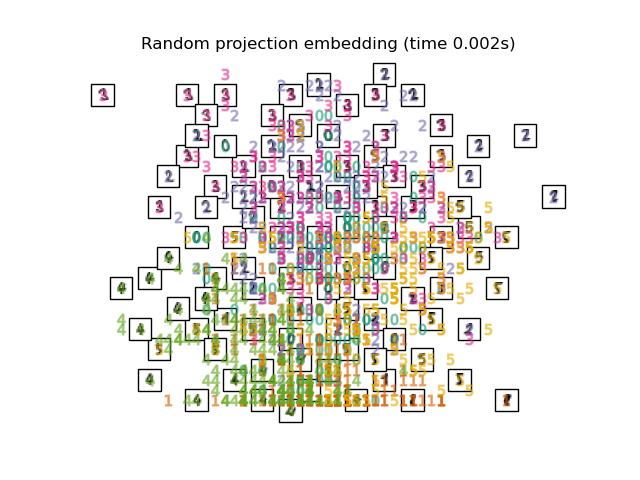•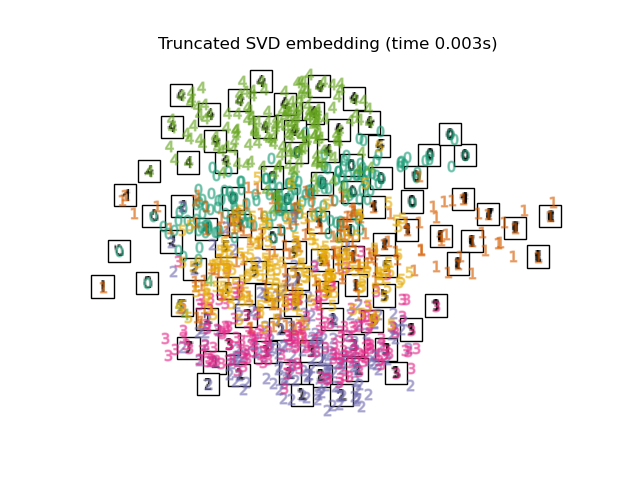•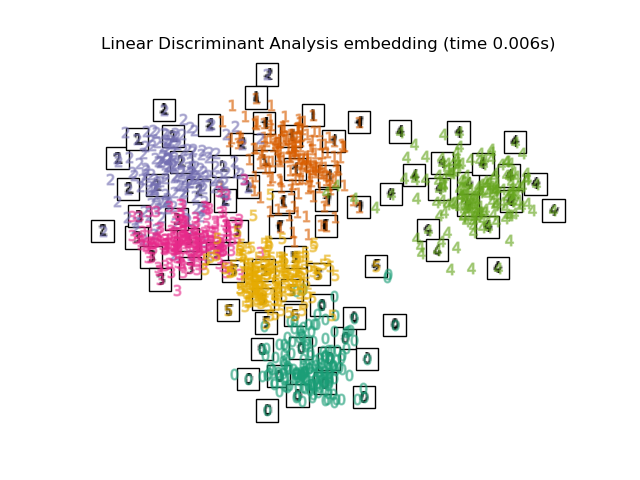•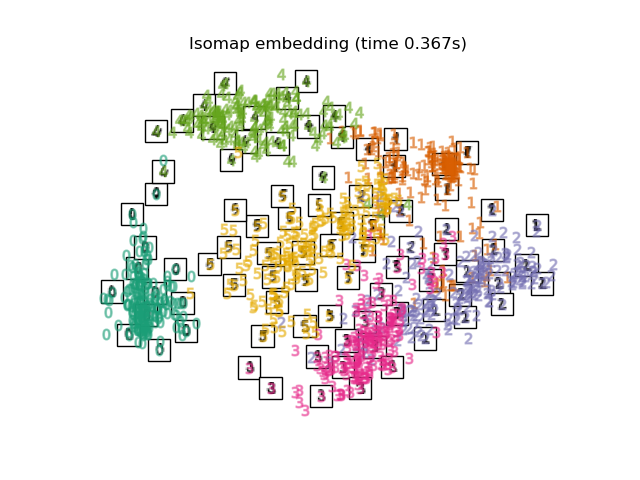•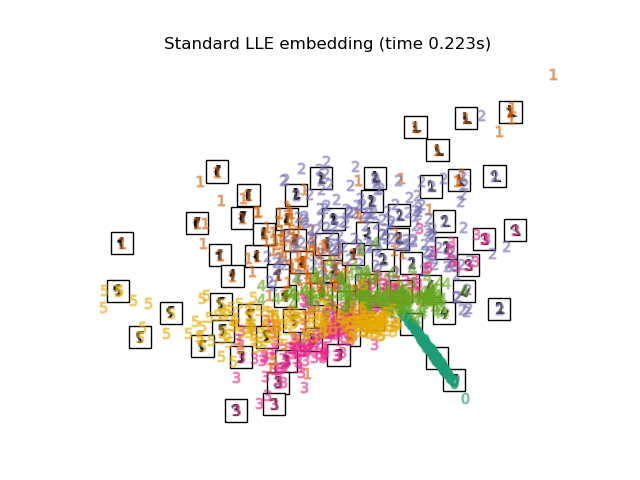•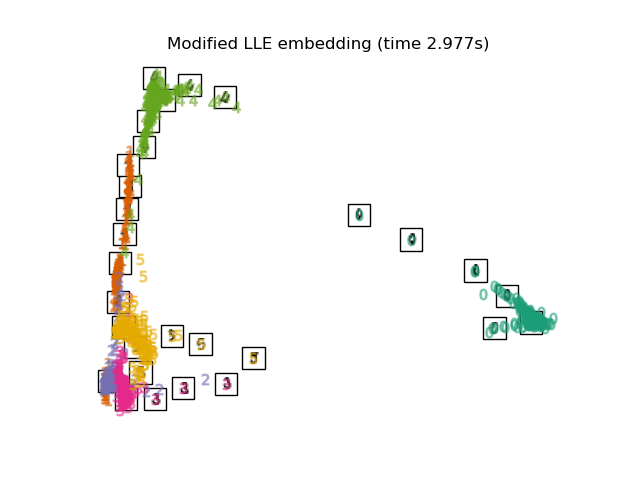•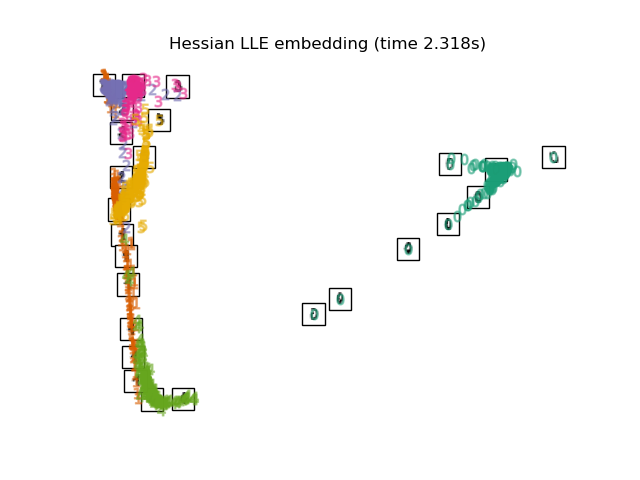•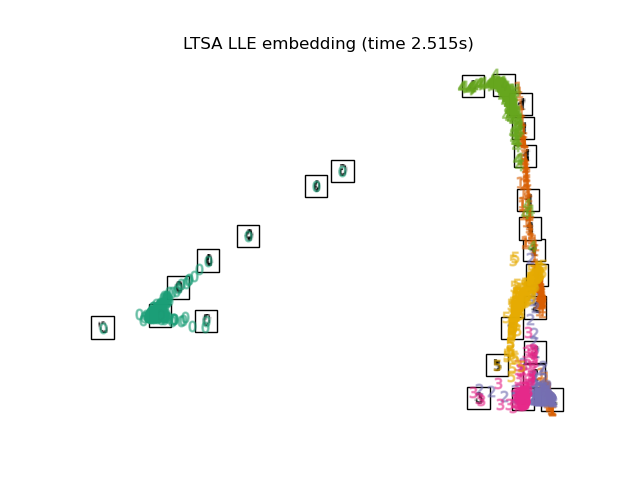•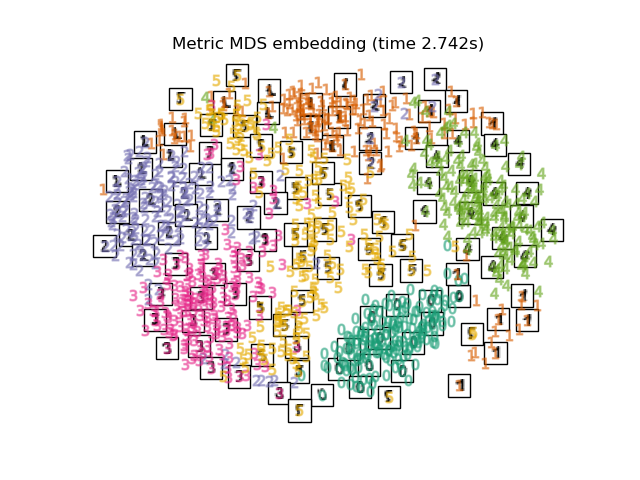•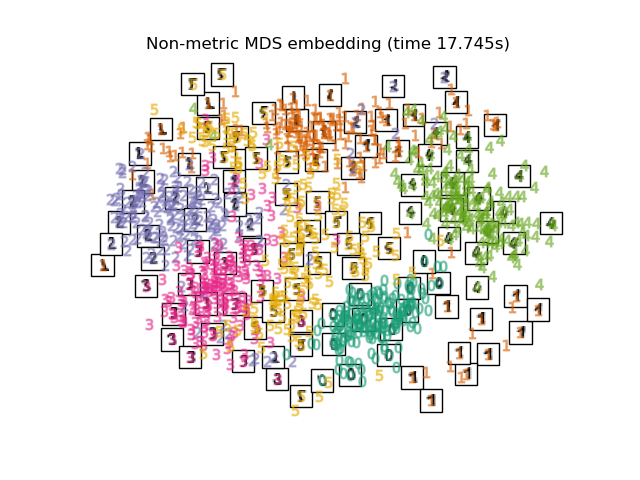•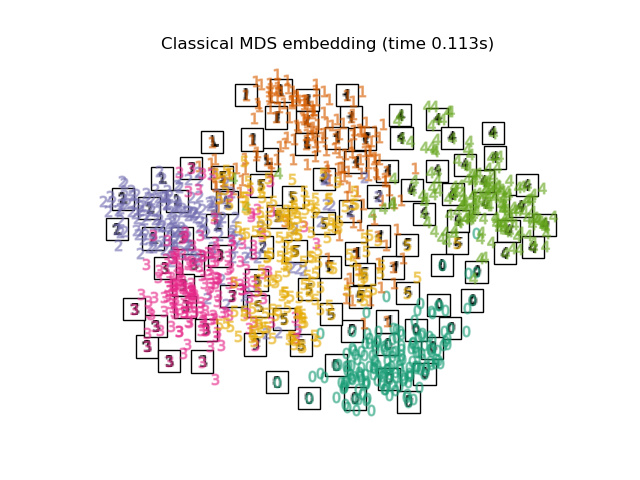•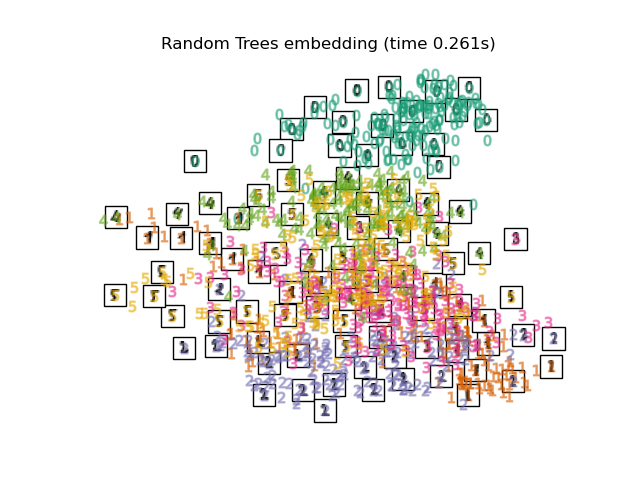•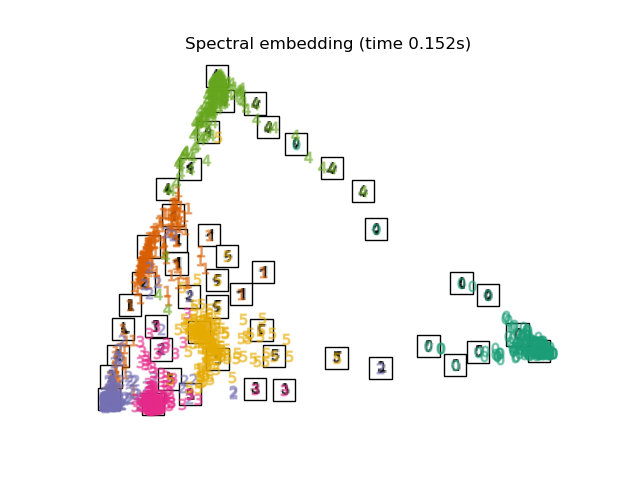Total running time of the script: (0 minutes 15.922 seconds)

Related examples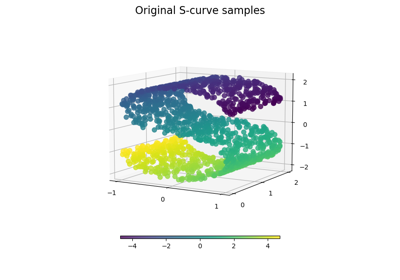Comparison of Manifold Learning methods

Comparison of Manifold Learning methods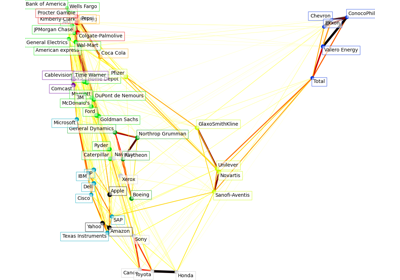Visualizing the stock market structure

Visualizing the stock market structure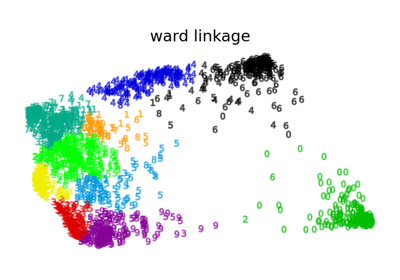Various Agglomerative Clustering on a 2D embedding of digits

Various Agglomerative Clustering on a 2D embedding of digits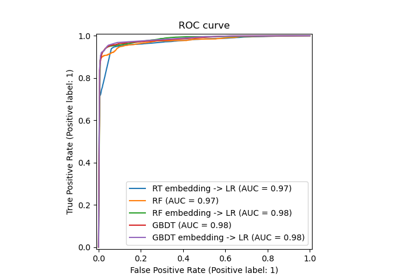Feature transformations with ensembles of trees

Feature transformations with ensembles of trees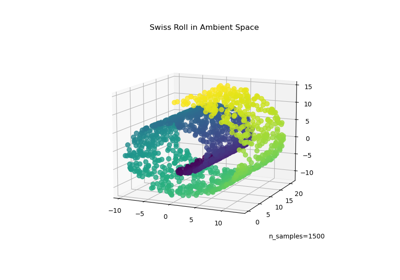Swiss Roll And Swiss-Hole Reduction

Swiss Roll And Swiss-Hole Reduction

Gallery generated by Sphinx-Gallery